# Colleges with the highest SAT scores in Maine

Top 10 colleges in Maine with the highest SAT scores
Looking for the colleges with the highest SAT scores in Maine? Well you're in luck! We've compiled a national college database and have created a list of the top 10 universities with the highest SAT scores in Maine. These are the schools whose applicants had the highest average SAT scores in Maine. And, since these tests are meant to determine academic prowess, they are arguably the schools with the most academically proficient students. You could even say these are the best colleges in Maine. We also include each college's ACT scores and acceptance rate so that you can see where you would have the easiest or hardest time getting in. Read on to find out more.

## Colby College SAT scores

The average SAT score for Colby College is 1430.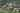The average SAT score of 1430 breaks down into:

• SAT math: 730

The average ACT score for Colby College is 32 and their acceptance rate is 13.1%.

## Bowdoin College SAT scores

The average SAT score for Bowdoin College is 1405.The average SAT score of 1405 breaks down into:

• SAT math: 710

The average ACT score for Bowdoin College is 32 and their acceptance rate is 10.3%.

## Bates College SAT scores

The average SAT score for Bates College is 1380.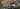The average SAT score of 1380 breaks down into:

• SAT math: 700

The average ACT score for Bates College is 31 and their acceptance rate is 17.8%.

## College of the Atlantic SAT scores

The average SAT score for College of the Atlantic is 1269.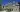The average SAT score of 1269 breaks down into:

• SAT math: 600

The average ACT score for College of the Atlantic is 28 and their acceptance rate is 67.9%.

## University of Maine SAT scores

The average SAT score for University of Maine is 1154.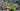The average SAT score of 1154 breaks down into:

• SAT math: 570

The average ACT score for University of Maine is 24 and their acceptance rate is 92.3%.

## University of New England SAT scores

The average SAT score for University of New England is 1127.The average SAT score of 1127 breaks down into:

• SAT math: 560

The average ACT score for University of New England is 24 and their acceptance rate is 80.7%.

## Maine Maritime Academy SAT scores

The average SAT score for Maine Maritime Academy is 1110.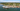The average SAT score of 1110 breaks down into:

• SAT math: 560

The average ACT score for Maine Maritime Academy is 22 and their acceptance rate is 78.6%.

## Unity College SAT scores

The average SAT score for Unity College is 1100.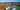The average SAT score of 1100 breaks down into:

• SAT math: 540

The average ACT score for Unity College is 22 and their acceptance rate is 88.7%.

## Husson University SAT scores

The average SAT score for Husson University is 1060.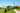The average SAT score of 1060 breaks down into:

• SAT math: 520

The average ACT score for Husson University is 20 and their acceptance rate is 80.2%.

## Saint Joseph's College of Maine SAT scores

The average SAT score for Saint Joseph's College of Maine is 1040.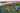The average SAT score of 1040 breaks down into:

• SAT math: 510